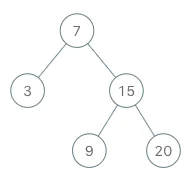## Problem

Implement an iterator over a binary search tree (BST). Your iterator will be initialized with the root node of a BST.

Calling next() will return the next smallest number in the BST.

Example:Note:

• next() and hasNext() should run in average O(1) time and uses O(h) memory, where h is the height of the tree.
• You may assume that next() call will always be valid, that is, there will be at least a next smallest number in the BST when next() is called.

O(N)

O(h)